# High School Chemistry : Types of Reactions

## Example Questions

2 Next →

### Example Question #3 : Help With Oxidation Reduction Reactions

What is the oxidation number of sulfur in the given compound?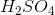Possible Answers: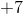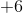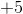Correct answer:Explanation:

Use the rules for assigning oxidation numbers. We know that the sum of oxidation numbers of all atoms present in the compound must equal zero because the compound is neutral.

The oxidation number of hydrogen is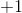except in compounds with less electronegative elements (which is not the case here). Because there are two hydrogens, the overall oxidation is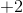.

The oxidation number of oxygen is always. Multiply that by the total number of oxygens to get.

From here, simple math tells us that the oxidation number of sulfur must beto make the total oxidation state equal to zero.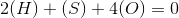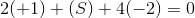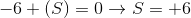### Example Question #1 : Help With Oxidation Reduction Reactions

Which of the following describes a high energy, exothermic redox reaction that produces carbon dioxide and water?

Possible Answers:

Nuclear

Acid-base

Decomposition

Combustion

Boiling

Correct answer:

Combustion

Explanation:

A combustion reaction is characterized as an oxidation-reduction reaction because the oxidation number of the reactants changes, and oxygen is used to burn a fuel molecule. Carbon dioxide is often produced as a product. The following is an example of a combustion reaction: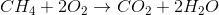### Example Question #5 : Help With Oxidation Reduction Reactions

Which of the following describes an oxidation-reduction reaction?

Possible Answers:

A type of reaction in which one reactant is broken down into two products

The transfer of electrons between species, and the subsequent changes in oxidation states

A reaction in which one species molecule is oxidized then reduced

None of these

A type of chemical reaction that always involves acids and bases

Correct answer:

The transfer of electrons between species, and the subsequent changes in oxidation states

Explanation:

In an oxidation reaction, one species is oxidized (loses electrons). During a reduction reaction, one species is reduced (gains electrons). These transfers of electrons results in the change of oxidation states. One way to help you remember which reaction is which, is by using the mnemonic: OIL RIG - Oxidation Is Loss of electrons; Reduction Is Gain of electrons.

2 Next →

### All High School Chemistry Resources